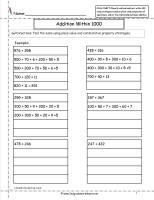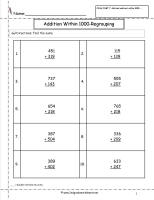﻿ CCSS 2.NBT.7 Worksheets, Addition and Subtraction Within 1000 Worksheets

# Common Core State Standard 2.NBT.7

## Number & Operations in Base Ten

### Use place value understanding and properties of operations to add and subtract.

7. Add and subtract within 1000, using concrete models or drawings and strategies based on place value, properties of operations, and/or the relationship between addition and subtraction; relate the strategy to a written method. Understand that in adding or subtracting three-digit numbers, one adds or subtracts hundreds and hundreds, tens and tens, ones and ones; and sometimes it is necessary to compose or decompose tens or hundreds.

###Addition Within 10002345

Common Core State Standards: 2.NBT.7
Number & Operations in Base Ten
Use Place Value Understanding and Properties of Operations to Add and Subtract.

Students complete addition problems using place value strategies.

###Addition Within 10002345

Common Core State Standards: 2.NBT.7
Number & Operations in Base Ten
Use Place Value Understanding and Properties of Operations to Add and Subtract.